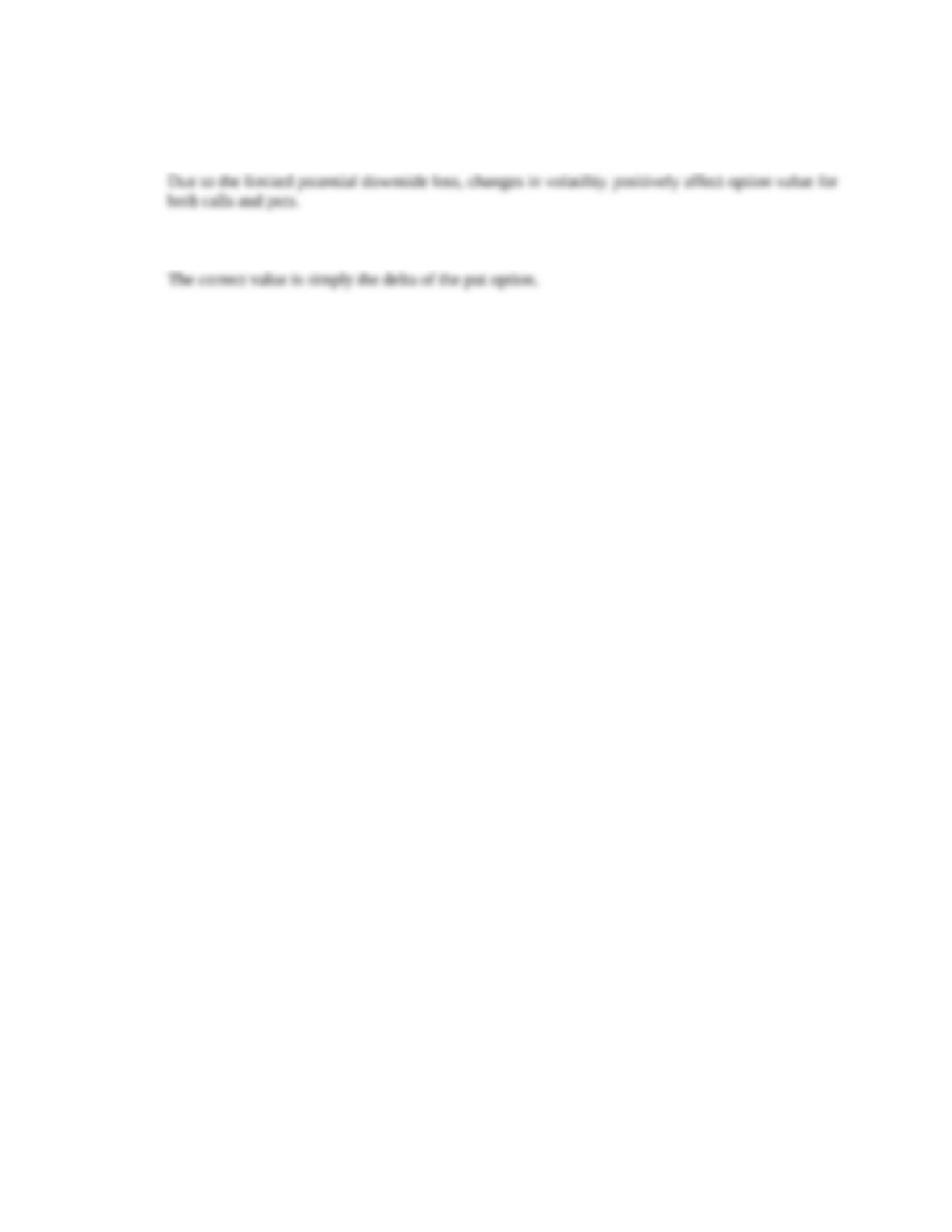Type
Solution Manual
Book Title
Fundamentals of Investments: Valuation and Management 8th Edition
ISBN 13
978-1259720697

### 978-1259720697 Chapter 16 Solution Manual

January 2, 2020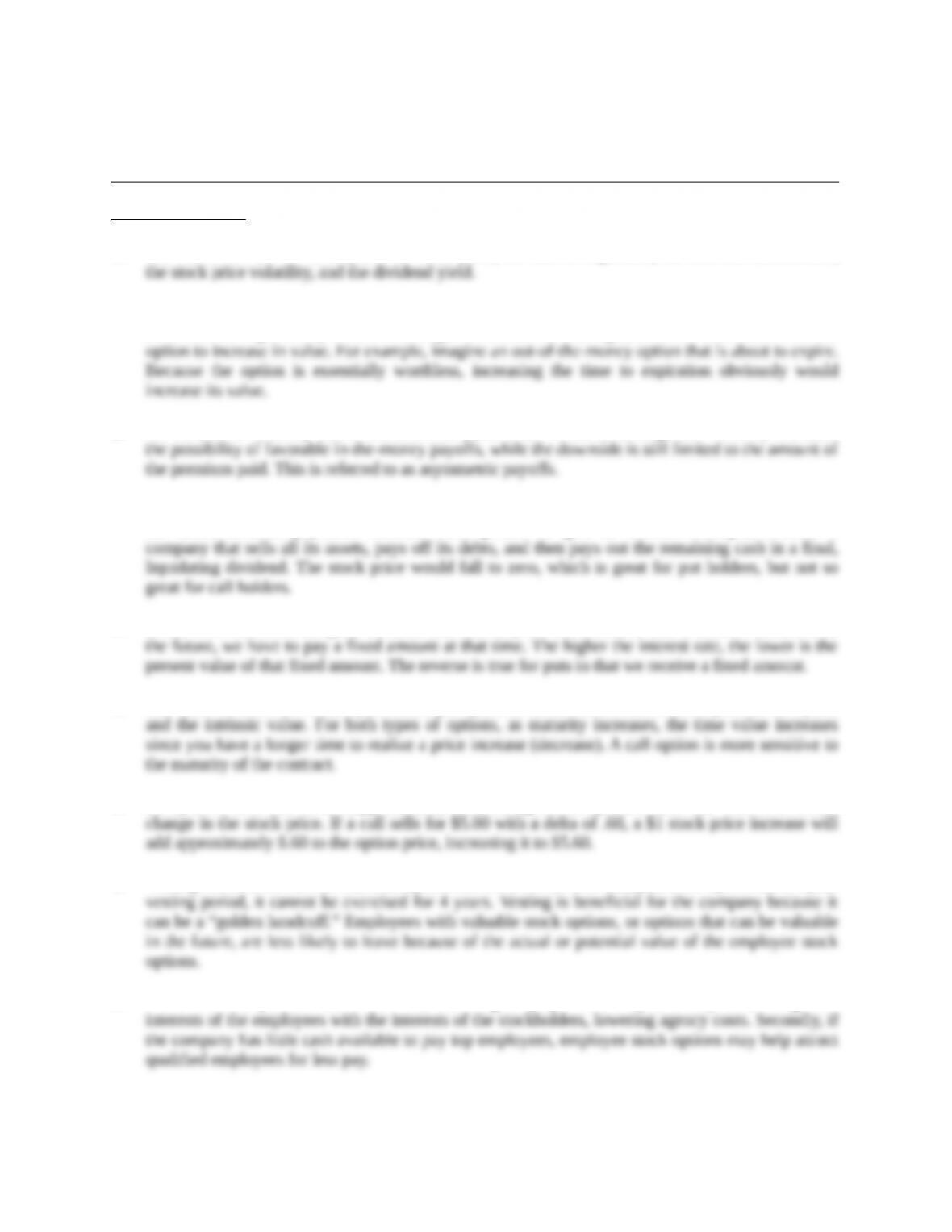Chapter 16
Option Valuation
Concept Questions
1. The six factors are the stock price, the strike price, the time to expiration, the risk-free interest rate,
2. Increasing the time to expiration increases the value of an option. The reason is that the option gives
the holder the right to buy or sell. The longer the holder has that right, the more time there is for the
3. An increase in volatility acts to increase both put and call values because greater volatility increases
4. An increase in dividend yield reduces call values and increases put values. The reason is that, all else
the same, dividend payments decrease stock prices. To give an extreme example, consider a
5. Interest rate increases are good for calls and bad for puts. The reason is that if a call is exercised in
6. The time value of both a call option and a put option is the difference between the price of the option
7. An option’s delta tells us the (approximate) dollar change in the option’s value that will result from a
8. Vesting refers to the date at which an option can be exercised. For example, if the option has a 4 year
9. There are two possible benefits. First, awarding employee stock options may better align the
Education.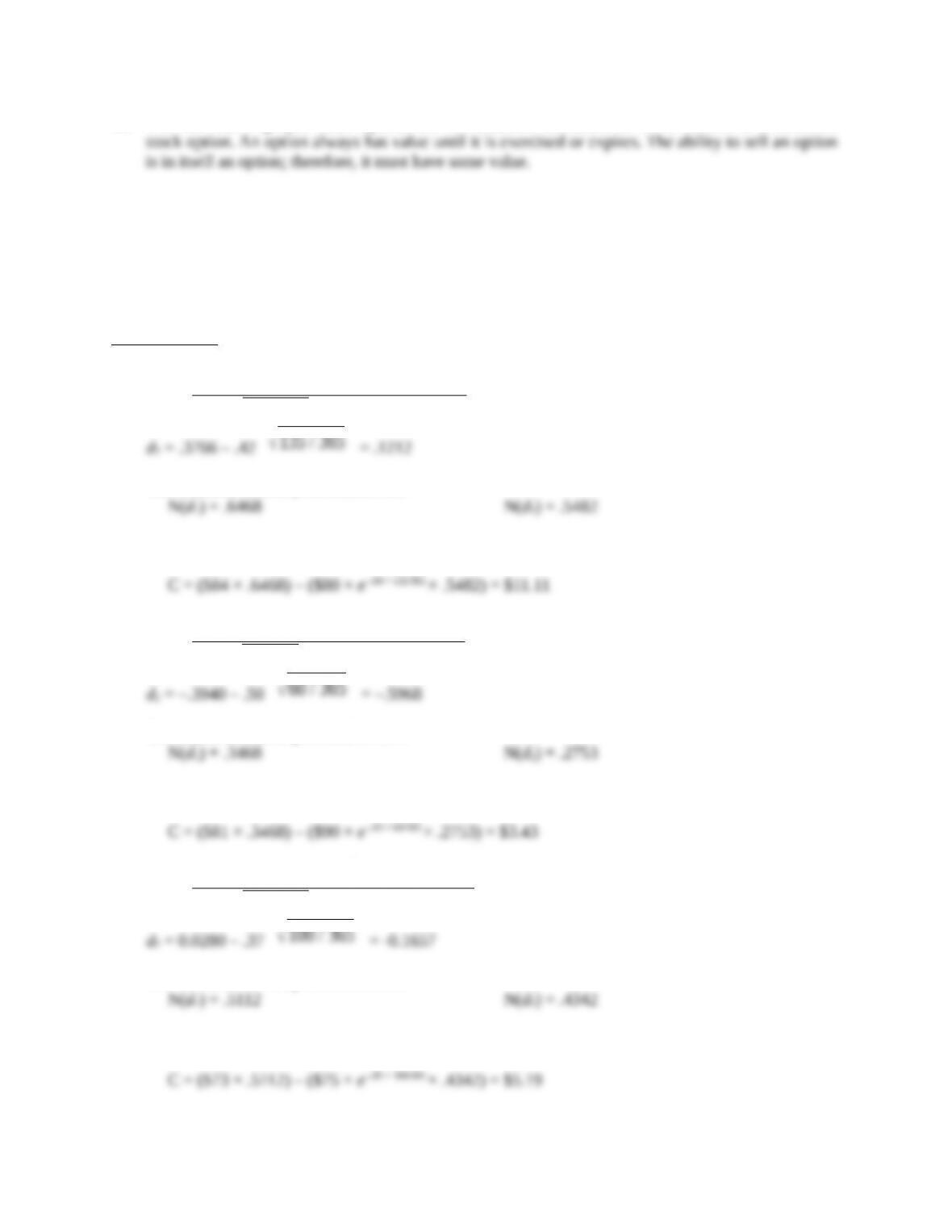10. The fact that employee stock options are not tradeable decreases its value relative to a tradeable
Solutions to Questions and Problems
NOTE: All end of chapter problems were solved using a spreadsheet. Many problems require multiple
steps. Due to space and readability constraints, when these intermediate steps are included in this
solutions manual, rounding may appear to have occurred. However, the final answer for each problem is
found without rounding during any step in the problem.
Core Questions
1. d1 =
The standard normal probabilities are:
Calculating the price of the call option yields:
2. d1 =
ln(81 / 90)+(.03 +. 502/ 2)× 60 / 365
.50 ×
60 / 365
= –.3940
The standard normal probabilities are:
Calculating the price of the call option yields:
3. d1 =
.37 ×
100 / 365
= .0280
The standard normal probabilities are:
Calculating the price of the call option yields:
Education.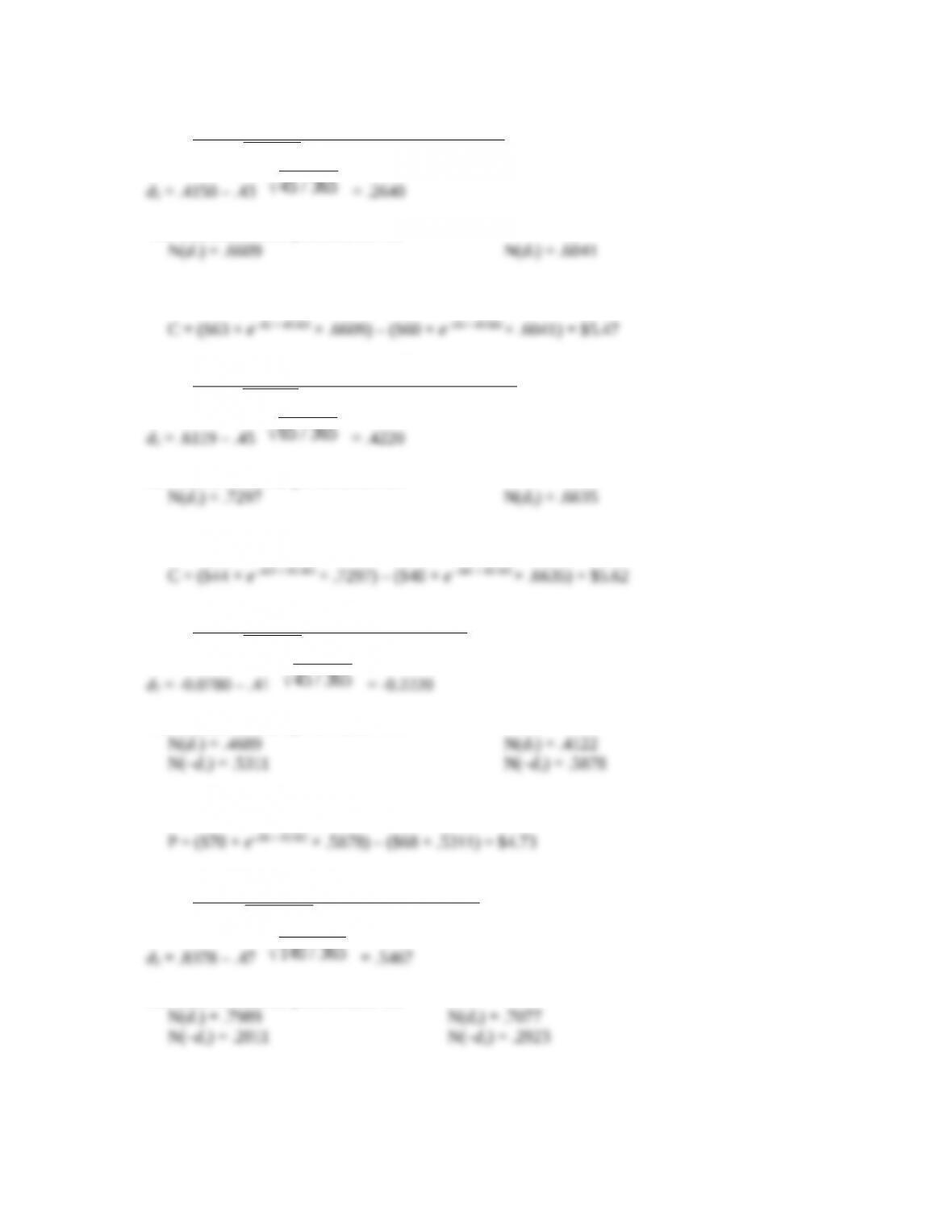4. d1 =
= .4150
The standard normal probabilities are:
Calculating the price of the call option yields:
5. d1 =
ln(44 / 40 )+(. 041 - .025 +. 452/ 2)× 65 / 365
. 45 ×
65 / 365
= .6119
The standard normal probabilities are:
Calculating the price of the call option yields:
6. d1 =
ln(68 / 70 )+(.06 +. 412/ 2 45 / 365
. 41 ×
45 / 365
= -0.0780
The standard normal probabilities are:
Calculating the price of the put option yields:
7. d1 =
ln(42 / 35 )+(. 05+. 472/ 2)× 140 / 365
. 47 ×
140 / 365
= .8378
The standard normal probabilities are:
Calculating the price of the put option yields:
Education.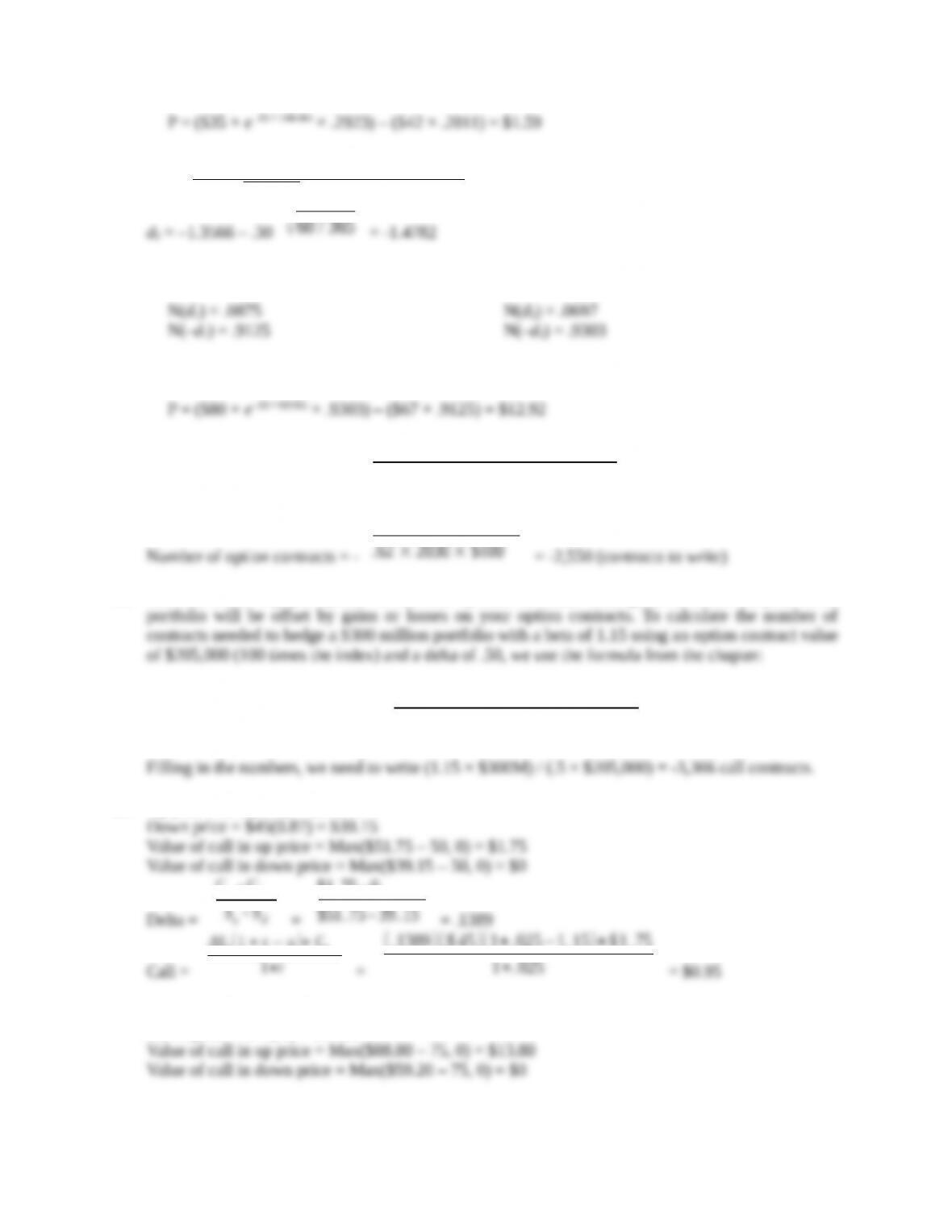8. d1 =
ln(67 / 80 )+(.03 +. 302/ 2 60 / 365
.30 ×
60 / 365
= –1.3566
The standard normal probabilities are:
Calculating the price of the put option yields:
9. Number of option contracts = -
Portfolio beta × Portfolio value
Option delta × Option contract value
Number of option contracts = -
10. You can either buy put options or sell call options. In either case, gains or losses on your stock
Number of option contracts = -
Portfolio beta × Portfolio value
Option delta × Option contract value
11. Up price = \$45(1.15) = \$51.75
Delta =
Cu - Cd
Su - Sd
=
Call =
ΔSu(1 + r u )+ Cu
1+r
=
12. Up price = \$74(1.2) = \$88.80
Down price = \$74(.8) = \$59.20
Education.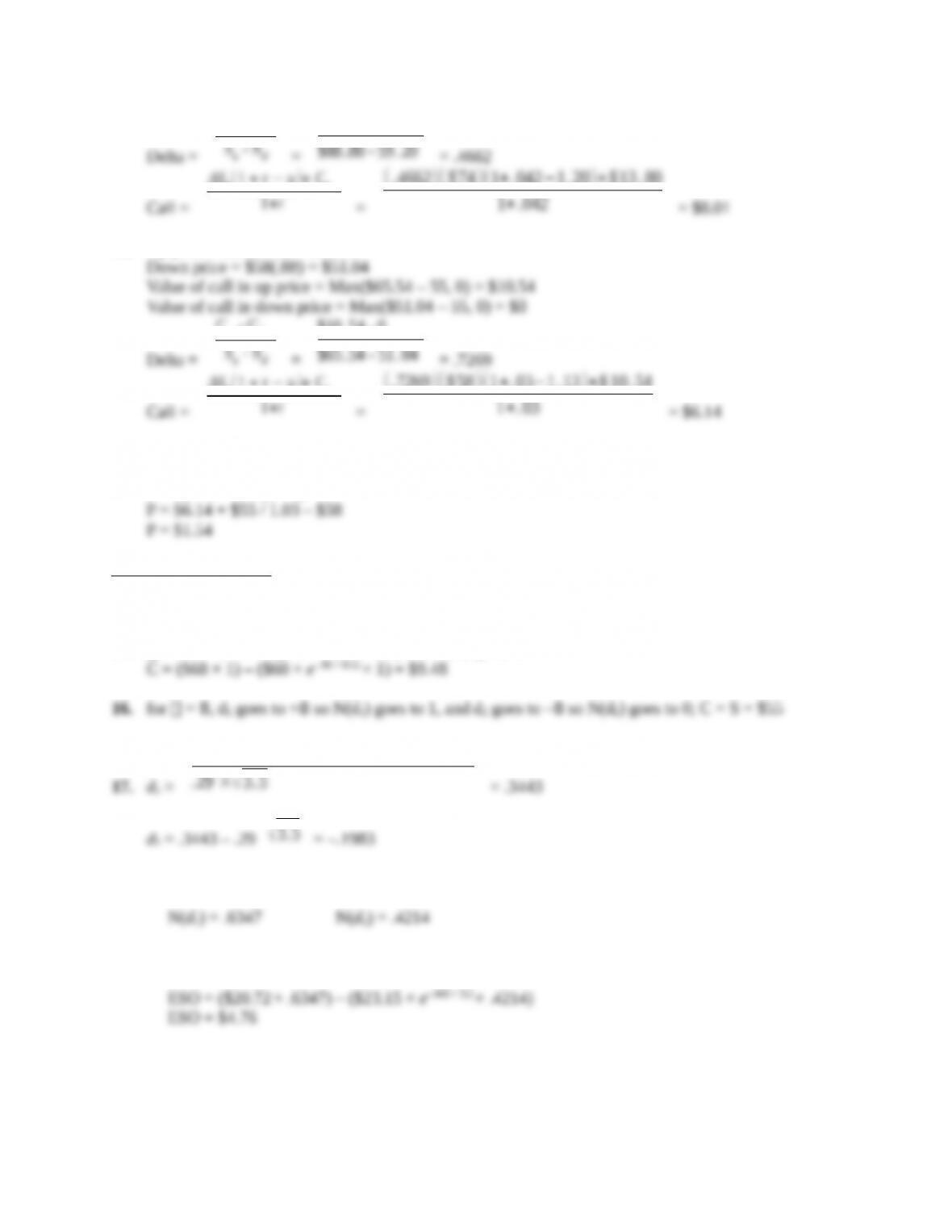Delta =
Cu - Cd
Su - Sd
=
Call =
ΔSu(1 + r u )+ Cu
1+r
=
13. Up price = \$58(1.13) = \$65.54
Delta =
Cu - Cd
Su - Sd
=
Call =
ΔSu(1 + r u )+ Cu
1+r
=
Using put-call parity:
P + S0 = C + K / (1 + r)
Intermediate Questions
14. K = 0, so C = S = \$70
15. = 0, so d1 and d2 go to +8, so N(d1) and N(d2) go to 1.
17. d1 =
These standard normal probabilities are given:
Calculating the price of the employee stock options yields:
18. This is a hedging problem in which you wish to hedge one option position with another. Your
employee stock option (ESO) position represents 10,000 shares, and you need to know how many
Education.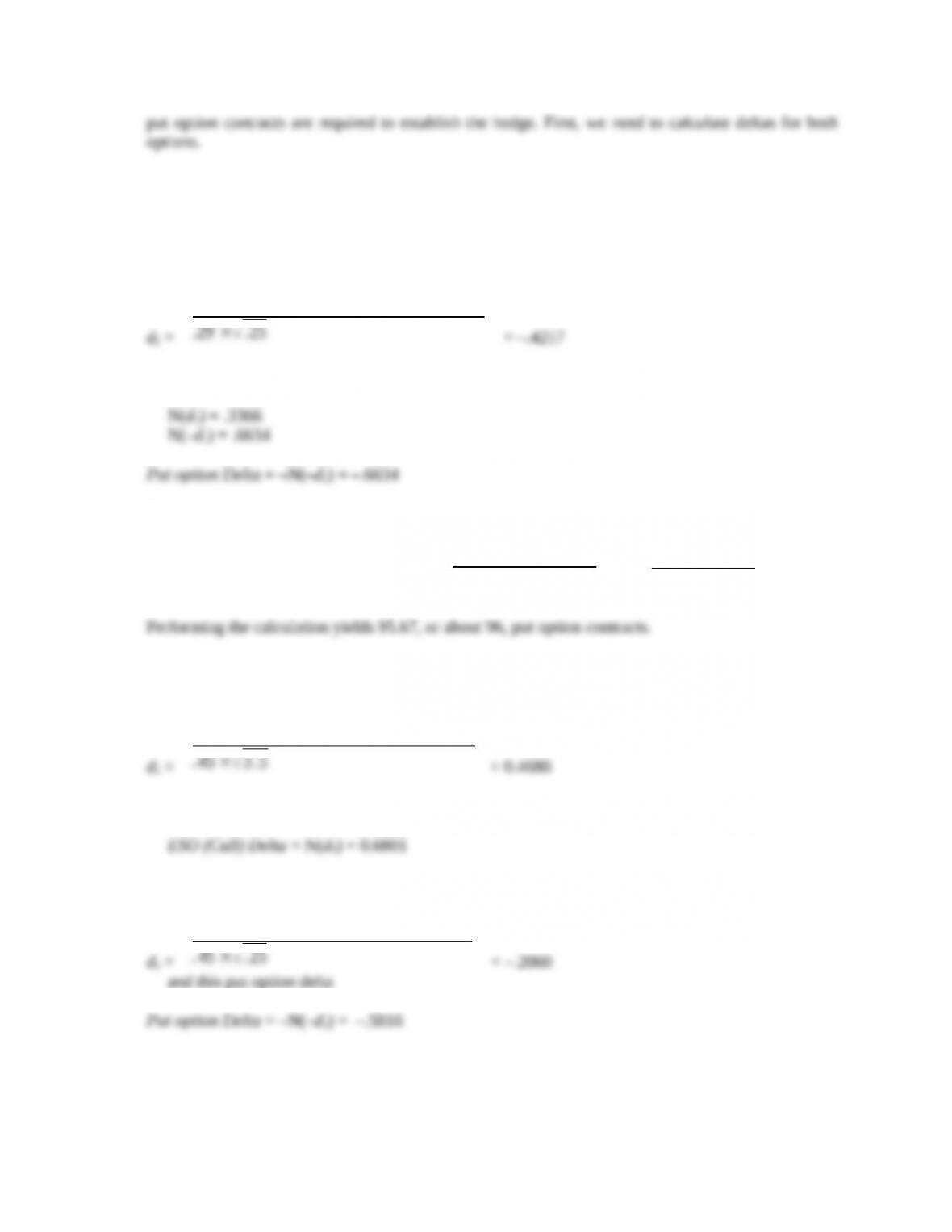Using values from the previous answer, the ESO delta is
ESO (Call) Delta = N(d1) = .6347
For the put option, we get this value for d1
d1 =
These standard normal probabilities are given:
The number of put option contracts is then calculated as
Number of option contracts = –
= –
19. After the volatility shift, we need to recalculate deltas for both options. The new value of d1 for
the ESO is:
d1 =
In turn, the new ESO delta is
For the put option, we obtain this value for d1
d1 =
and this put option delta
The new number of contracts required is:
Education.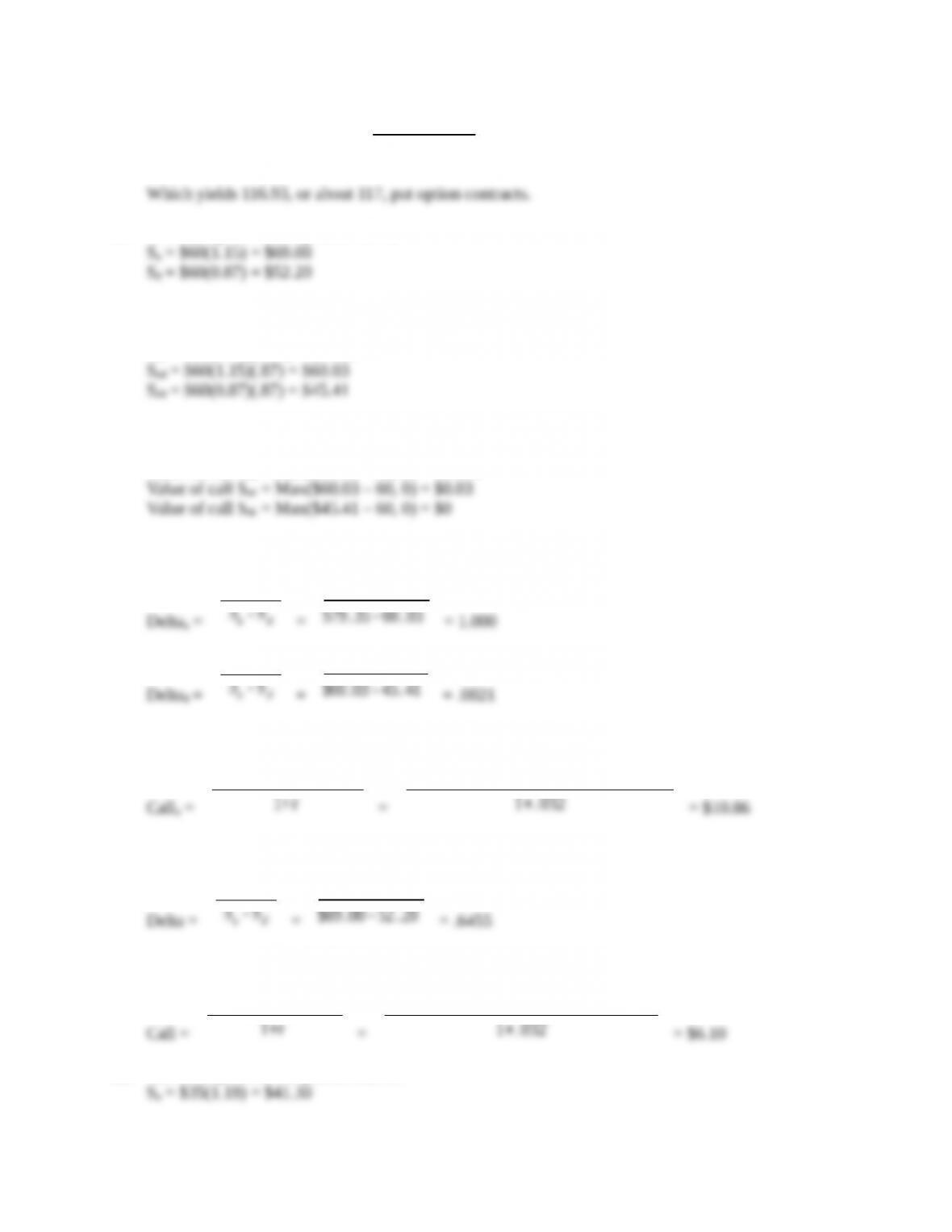Number of option contracts = -
20. The stock price in one period will be:
In two periods, the stock price will be:
Suu = \$60(1.15)(1.15) = \$79.35
The call value for each node is:
Value of call Suu = Max(\$79.35 – 60, 0) = \$19.35
The delta of the up and down moves will be:
Deltau =
Cu - Cd
Su - Sd
=
Cu - Cd
Su - Sd
=
So the call value after an up move will be:
Callu =
ΔSu(1 + r u )+ Cuu
1+r
=
The value of a call with a first down move is \$0 since it will always be worthless. The delta today is:
Delta =
Cu - Cd
Su - Sd
=
So, the value of call today is:
Call =
ΔS(1 + r u )+ Cu
1+r
=
21. The stock price in one period will be:
Education.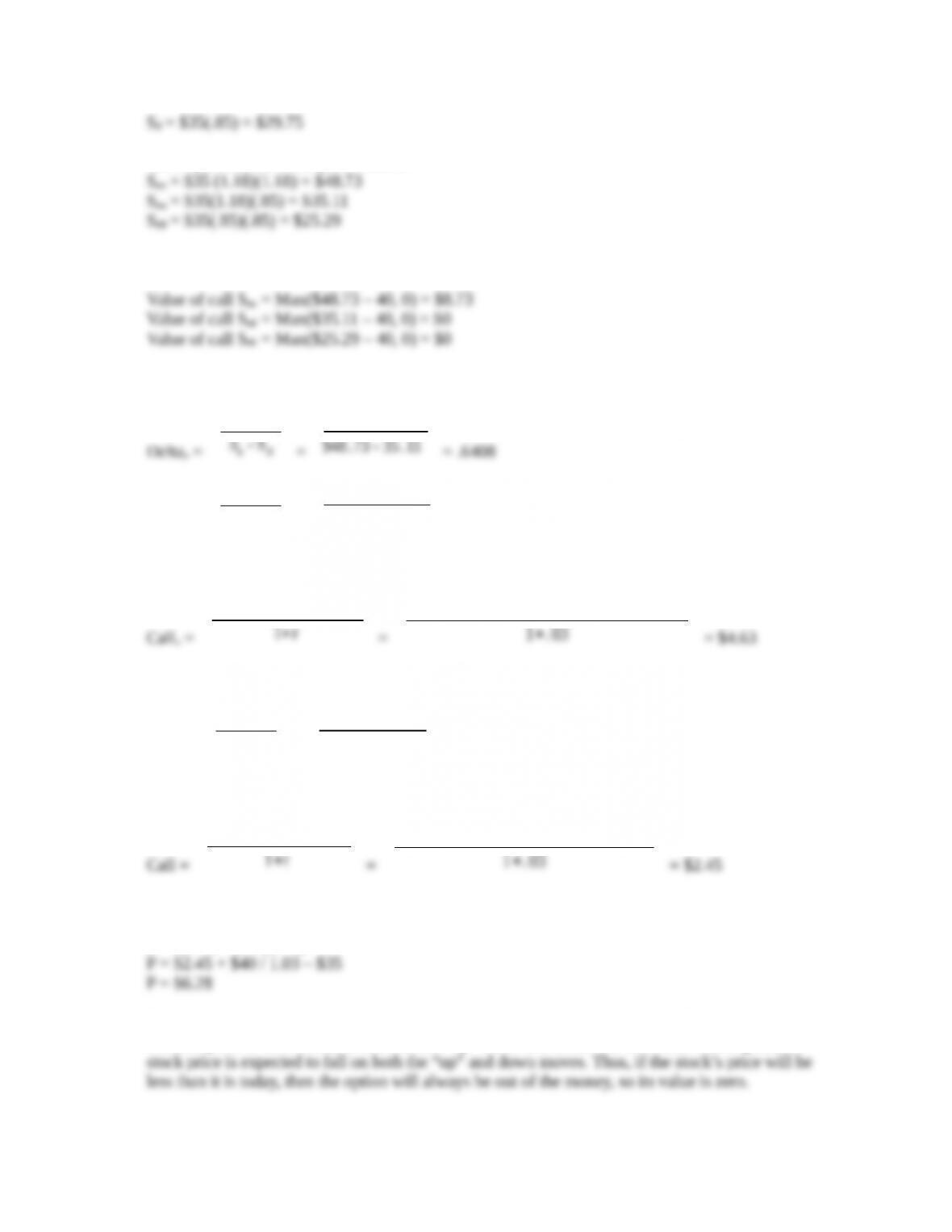In two periods, the stock price will be:
The call value for each node is:
The delta of the up and down moves will be:
Deltau =
Cu - Cd
Su - Sd
=
Cu - Cd
Su - Sd
=
= 0
So the call value after an up move will be:
Callu =
ΔSu(1 + r u )+ Cuu
1+r
=
The value of a call with a first down move is \$0 since it will always be worthless. The delta today is:
Delta =
Cu - Cd
Su - Sd
=
= .4005
So, the value of call today is:
Call =
ΔS(1 + r u )+ Cu
1+r
=
Using put-call parity:
P + S0 = C + K / (1 + r)
Education.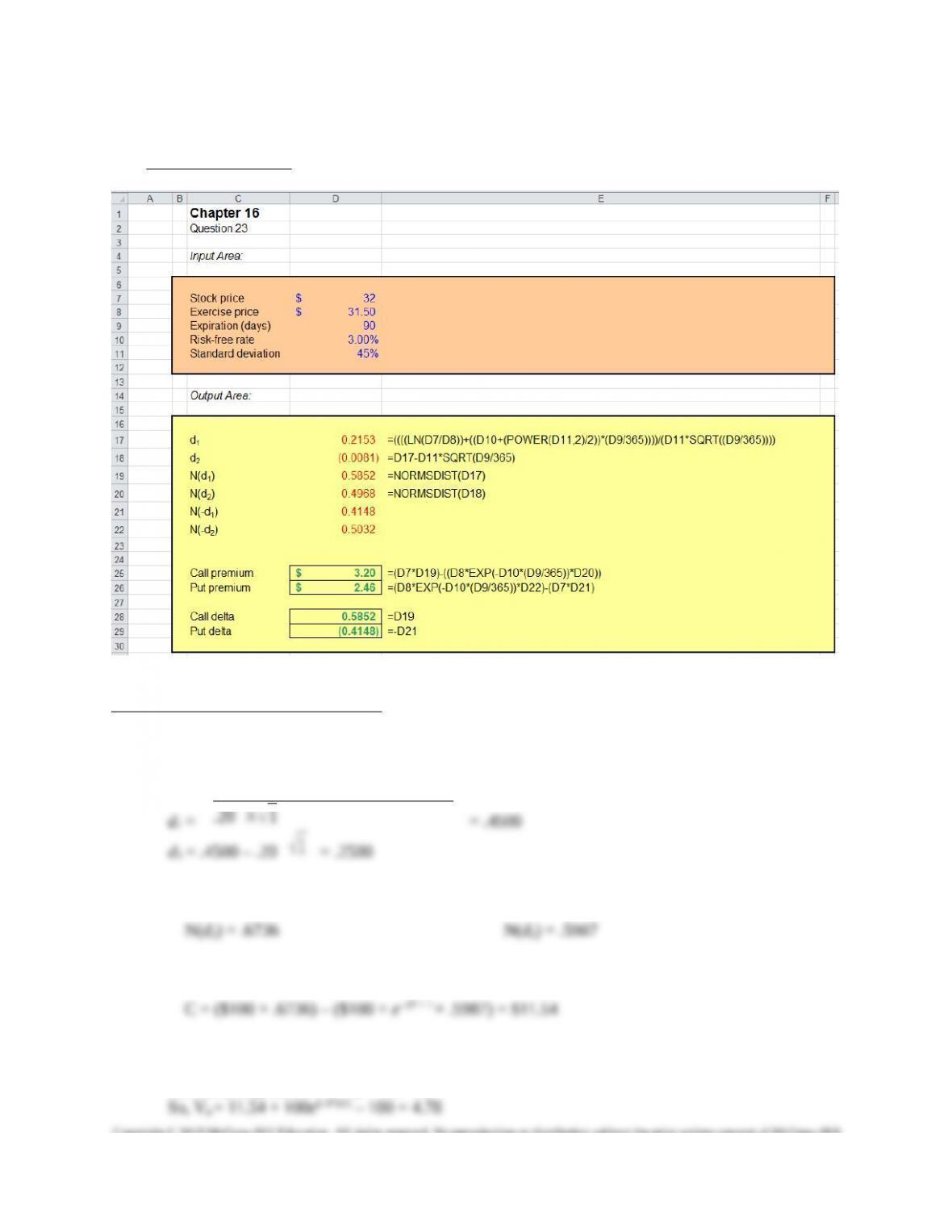CFA Exam Review by Kaplan Schweser
1. c
d1 =
1
The standard normal probabilities are:
Calculating the price of the call option yields:
2. a
Put-call parity states: S + Vp = Vc + Xe-rt